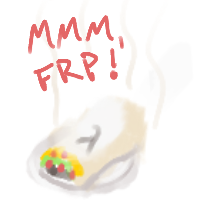Table of Ingredients

## Discovery

FRP has been around for a long time. Originally discovered and coined by Conal Elliot and Paul Hudak in the late 90’s it represented a new approach to writing dynamic systems, wherein the programmer describes how the system changes over time using continuous semantics. What this meant practically is that FRP code tended to look more like interelated equations and less like a list of steps to achieve a goal.

As time went on more implementations were made and the term got thrown around freely, eventually even being applied to other technologies like Javascript’s React framework. These days when folks talk about React, bacon.js, etc. they tend to drop the “functional” part of FRP in favor of “reactive”, which suits the nature of those frameworks better. Check out the wikipedia article on FRP for more info on what it is, specifically

## Prep Work

An FRP system deals in terms of continuous values and discrete events. Continuous values are often referred to as `Behavior`s while discrete events are simply … you guessed it … `Event`s! What this means is that your values always come wrapped in these contexts. For this reason I’ve been known to call writing FRP code as “advanced burrito making”. The newly popular (as of the first writing of this article) reflex package adds another context - one that combines `Behavior`s and `Event`s, called `Dynamic`s. Reflex’s brand of FRP is “very advanced burrito making” ;)

In any implementation there are lots of functions for turning a value in one context into a value in another context … so if you are going to write in FRP I would recommend being really, really familiar with the basic typeclasses:

and if you’ve got the time it would really help to get these bad boys in as well -

Then once you have a grasp on the supporting machinery we can talk a bit about `Behavior`s and `Event`s.

## Behaviors

I find it beneficial to think of a `Behavior` as a function - like a mathematical function from your last physics class. Have you taken a physics class? No worries! This is just algebra!

Let’s use Newton’s law of gravitation, `f = g * (m1 * m2) / r ** 2`, where `f` is the force between two bodies of mass `m1` and `m2` respectively, `g` is a gravitational constant, which is just a number, and `r` is the distance between the centers of the two bodies. In a `Behavior`al context we can express this equation quite simply and quite literally by saying that if each term (`g`, `m1`, `m2` and `r`) are `Behavior`s themselves, then `f` is also a `Behavior`. Even better, if the FRP lib you choose defines Num and Fractional instances for its `Behavior` type then we can define `f` as the equation itself:

``f g m1 m2 r = g * (m1 * m2) / r ** 2``

In this case `g` is a constant, and due to `Fractional`’s fromRational we should be able to construct a `Behavior` for `g` simply using a float literal:

``f m1 m2 r = 6.674e−11 * (m1 * m2) / r ** 2``

So let’s say the bodies we’re finding the force between are planets. Then let’s exclaim “we have a type for that!”:

``````data Planet = Planet { planetMass     :: Float
, planetPosition :: V2 Float -- this is just a vector like (Float, Float), see the linear package
}

planetA = Planet 10 \$ V2 0 0
planetB = Planet 100 \$ V2 250 250``````

POOF! Planetary formation. Now we need to know how these planets change over time. Oooor we can assume they don’t. Either way we need a planet in a `Behavior`al context. `Behavior`s are often (always?) `Applicative`s. They have a first order kind (or more) like `* -> *`. In plain terms the `Behavior` type takes another type and wraps it in its context. This is `Functor` and `Applicative` stuff. So a planet in a `Behavior`al context would be something like `Behavior Planet`. Up until now I’ve left off the type variable in `Behavior`, but it should really be `Behavior a`, where `a` is any type.

Now, since many (all?) FRP implementations provide an `Applicative` instance for their `Behavior a` type, we can construct some `Behavior Planet`s:

``````planetBehaviorA = pure planetA
planetBehaviorB = pure planetB``````

These planet’s don’t change over time. These rocks just sit. Which reminds me of a poem:

Nobody sits like this rock sits. You rock, rock. The rock just sits - and is. You show us how to just sit here And that’s what we need. – Albert Markovski

Now that we have what we need (a couple of sitty rocks) we can write the other `Behavior`s we need, using `Functor` to great lengths … and masses.

``````posBehaviorA  = fmap planetPos planetBehaviorA
posBehaviorB  = fmap planetPos planetBehaviorB
massBehaviorA = fmap planetMass planetBehaviorA
massBehaviorB = fmap planetMass planetBehaviorB``````

Great! Now, we have `Behavior (V2 Float)`s for the planet’s positions over time, but what we need is the vector between them. We’re going to assume that `V2` has a `Num` instance (and it does, if we’re talking about this `V2`). Assuming this means we can treat it just like any other number because remember `Behavior a` also has a `Num` instance, at least for `a`s that have a `Num` instance (in this case `V2`) … so:

``vectorAB = posBehaviorA - posBehaviorB``

And that’s enough to get us the rest of the way:

``gForceAB = f massBehaviorA massBehaviorB vectorAB``

## A tour of FRP flavors

Evan Czaplicki of Elm fame did a great talk on the different flavors of FRP, and he does a better job describing them than I could:

## Wrap up that FRP-rrito

Hopefully that gives a little background to the situation. FRP has come a long way since 1997 but it’s really still an area of active research. Stay tuned for the next installment where we’ll look at some more Haskell code.